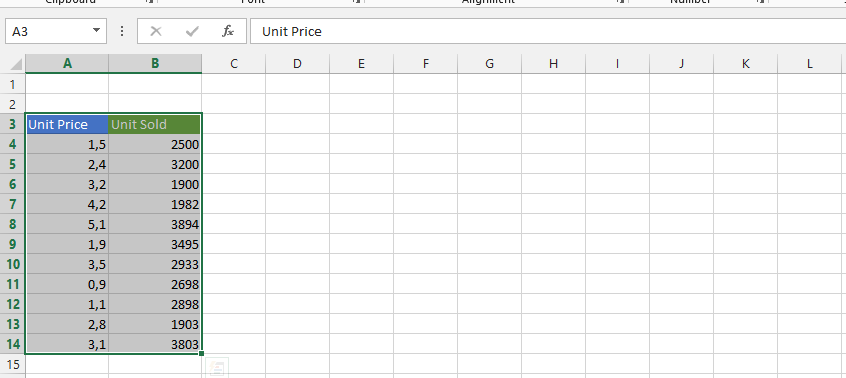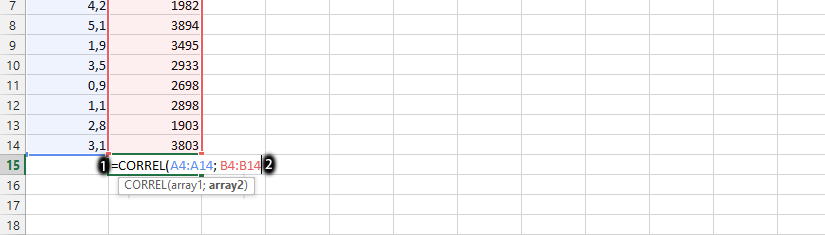#### How to calculate Modal?

The modal is the number that most often appears. We are going to learn how to calculate the modal with these simple steps.

## Data preparation

In Excel, you can calculate the mode by using the “MODE” function. Here’s how:

Open Microsoft Excel and enter your data into a column.Note: The spread numbers should have at least 2 numbers that are the same.

## Modal calculations

Click on a blank column, and type in =MODE(choose all the columns with numbers, ex. a5:a15), and then press enter. In this case, 4 is the modal.Modal formula in this example is =MODE(A1:A10)

Note that if your data has multiple modes, the “MODE” function will return the first mode it finds. If your data does not have any modes (i.e., if all values are unique), the “MODE” function will return the #N/A error.

In such cases, you can use the “MODE.MULT” function to calculate all the modes in a set of data. This function returns an array with all the modes.